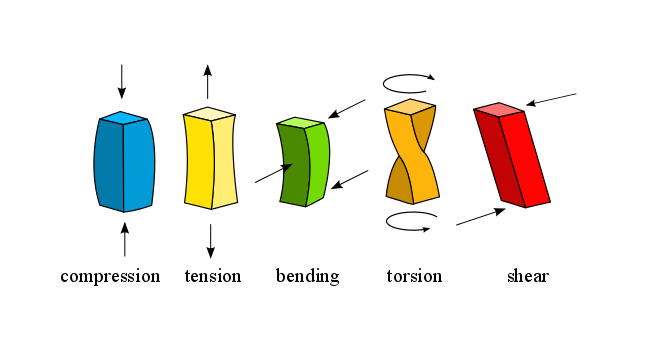ELEMENTARY FORCES

All of the forces in the universe are based on four fundamental interactions or elementary forces:

• Gravitational force: attraction force between 2 masses (m1, m2)
• Nuclear force: attraction force that keeps protons & neutrons together within the atom nucleus. Acts only at very short distances.
• Electric force: attraction or repulsion force between electric charges (++,--,+-,-+)
• Magnetic force: attraction or repulsion force between the poles of magnets or magnetized particles (NN, SS, NS, SN)

Of these forces, the gravitational force is the weakest.

In science, a field is a region of space where certain forces are present, so we talk about gravitational fields, electric fields, magnetic fields.

Due to the relationship between electricity and magnetism, it is common to talk about electromagnetism, electromagnetic fields and electromagnetic forces.

APPLIED FORCE

Apart from the elementary forces which are always present, we are free to apply a force on any object, at any time.

In physics, a force is any interaction that, when unopposed, will change the motion of an object. However, very often objects are not free to move, since they have simultaneous forces acting on them. Instead of moving, those objects suffer a certain "internal mechanical stress". See below an example of each type:COMBINED FORCES

We talk about combined forces when we have 2 or more forces acting simultaneously on an object. If those forces work in opposite directions, the object cannot move, and it suffers "internal mechanical stress". We can identify the following types of combined forces.

 COMBINED FORCES Example of object  bearing this type of force Example of structure (rod) bearing this type of force COMPRESSION the legs of your table Strut TENSION the string which hold the ceiling lamp Tie BENDING a diving board Beam TORSION a drill or a screwdriver Axle or shaft SHEAR a paper being cut by a pair of scissors Beam where it is supported by the wallThe bending force can be seen as COMPRESSION at the top + TENSION at the bottom.APPLIED FORCE on a free moving object

In physics, a force is any interaction that, when unopposed, will change the motion of an object. A force can cause an object with mass to change its velocity (which includes to begin moving from a state of rest), i.e., to accelerate. Force can also be described intuitively as a push or a pull. A force has both magnitude and direction, making it a vector quantity. It is measured in the SI unit of Newtons and represented by the symbol F.

One newton is the force needed to accelerate one kilogram of mass at the rate of one metre per second squared in direction of the applied force.F = m x a

where

• f (N or Newtons)
• m (kg)
• a (m/s2)

Example: calculate the force of gravity (aka weight) assuming m=70kg and ag=10m/s2

F (N) = m (kg) x ag (m/s2)

= 70 x 10 = 700 N

Concepts related to force include: thrust, which increases the velocity of an object; drag, which decreases the velocity of an object; and torque, which produces changes in rotational speed of an object. In an extended body, each part usually applies forces on the adjacent parts; the distribution of such forces through the body is the internal mechanical stress. Such internal mechanical stresses cause no accelation of that body as the forces balance one another.